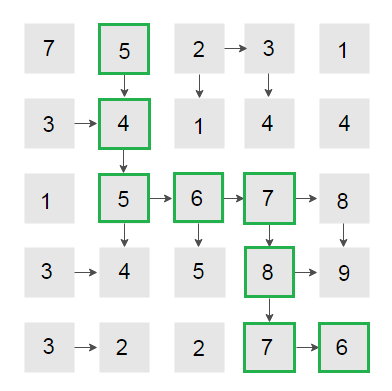# Maximum Length Snake Sequence

Given a square matrix, print maximum length snake sequence in it. A Snake sequence is defined as a sequence of numbers where each new number, which can only be located to the right or down of the current number, is either plus or minus one.

For example, from a given cell in a matrix, we can either move right (if that number is ± 1) or move down (if that number is ± 1). The goal of the problem is to find the longest path (Snake Sequence) through the matrix keeping in mind we can only move to a new cell whose value is ± 1 with respect to the current cell.

For example, Maximum length Snake sequence for below matrix is 5 – 4 – 5 – 6 – 7 – 8 – 7 – 6 as highlighted belowPlease note that multiple maximum length snake sequences can exist in the matrix. For example, 3 – 4 – 5 – 6 – 7 – 8 – 7 – 6 is another maximum length snake sequence in above matrix.

We can use Dynamic Programming to solve this problem. For each matrix cell (i, j), we need to calculate maximum length of a snake (say L[i][j]) which ends in that cell. Recurrence relation for calculating L[i][j] will be –

if (abs(M[i][j] – M[i][j – 1]) == 1)
L[i][j] = max(L[i][j], L[i][j – 1] + 1)

if (abs(M[i][j] – M[i – 1][j]) == 1)
L[i][j] = max(L[i][j], L[i – 1][j] + 1)

Now, the cell having the maximum value will correspond to the tail of the snake and we can easily trace path back to the head of the snake using L[][] matrix.

## C++

Output:

Maximum length Snake sequence : 5 – 4 – 5 – 6 – 7 – 8 – 7 – 6
Length is : 7

## Java

Output:

Maximum length Snake sequence : 5 – 4 – 5 – 6 – 7 – 8 – 7 – 6
Length is : 7

The time complexity of above solution is O(N*N) and auxiliary space used by the program is O(N*N).

Exercise: Extend the solution for a rectangular matrix(2 votes, average: 5.00 out of 5)Loading...

Please use our online compiler to post code in comments. To contribute, get in touch with us.
Like us? Please spread the word and help us grow. Happy coding 🙂Subscribe
Notify ofGuest
arandomguy

3-4-5-6-7-8-7-6 is not an example because in the snake sequence one can move only right or down.Guest
arandomguy

Also length should path.size() , i.e. 8 and not 7.Guest

This solution is incomplete.
You need to construct 4 such matrices, each starting at a different corner of the grid.
the result is the max path from 4 of the matrices.
consider the following grid:
1 2 3
2 0 0
3 0 0
the maximum snake is 3-2-1-2-3
but the above solution does not find it because it starts at the top left corner and moves down and right.
if you start at the top right and move down and left, you will find this path.